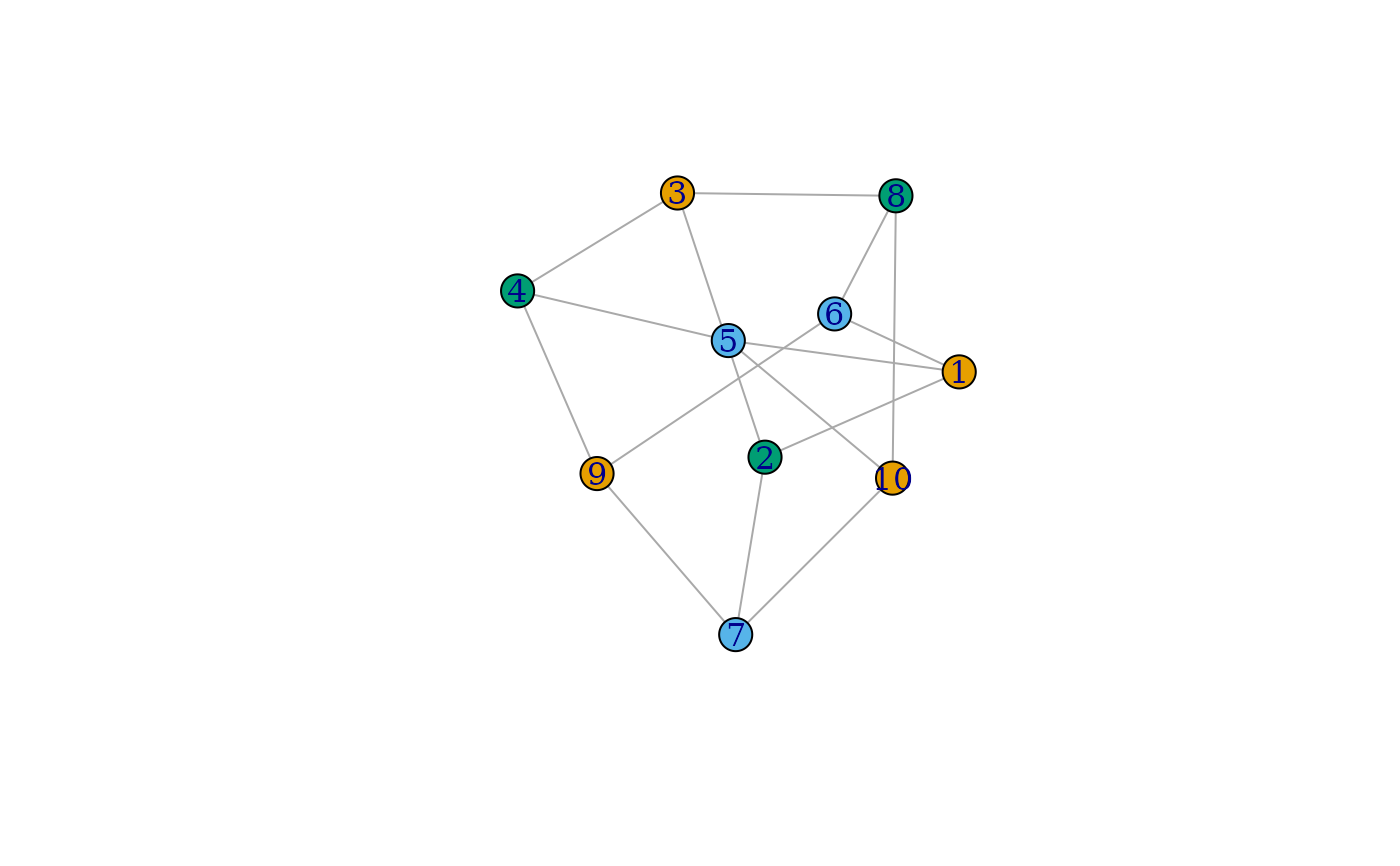greedy_vertex_coloring() finds a coloring for the vertices of a graph based on a simple greedy algorithm.

## Usage

greedy_vertex_coloring(graph, heuristic = c("colored_neighbors", "dsatur"))

## Arguments

graph

The graph object to color

heuristic

The selection heuristic for the next vertex to consider. Currently only one heuristic is supported: “colored_neighbors” selects the vertex with the largest number of already colored neighbors.

## Value

A numeric vector where item i contains the color index associated to vertex i

## Details

The goal of vertex coloring is to assign a "color" (i.e. a positive integer index) to each vertex of the graph such that neighboring vertices never have the same color. This function solves the problem by considering the vertices one by one according to a heuristic, always choosing the smallest color index that differs from that of already colored neighbors. The coloring obtained this way is not necessarily minimal but it can be calculated in linear time.

## Examples


g <- make_graph("petersen")
col <- greedy_vertex_coloring(g)
plot(g, vertex.color = col)